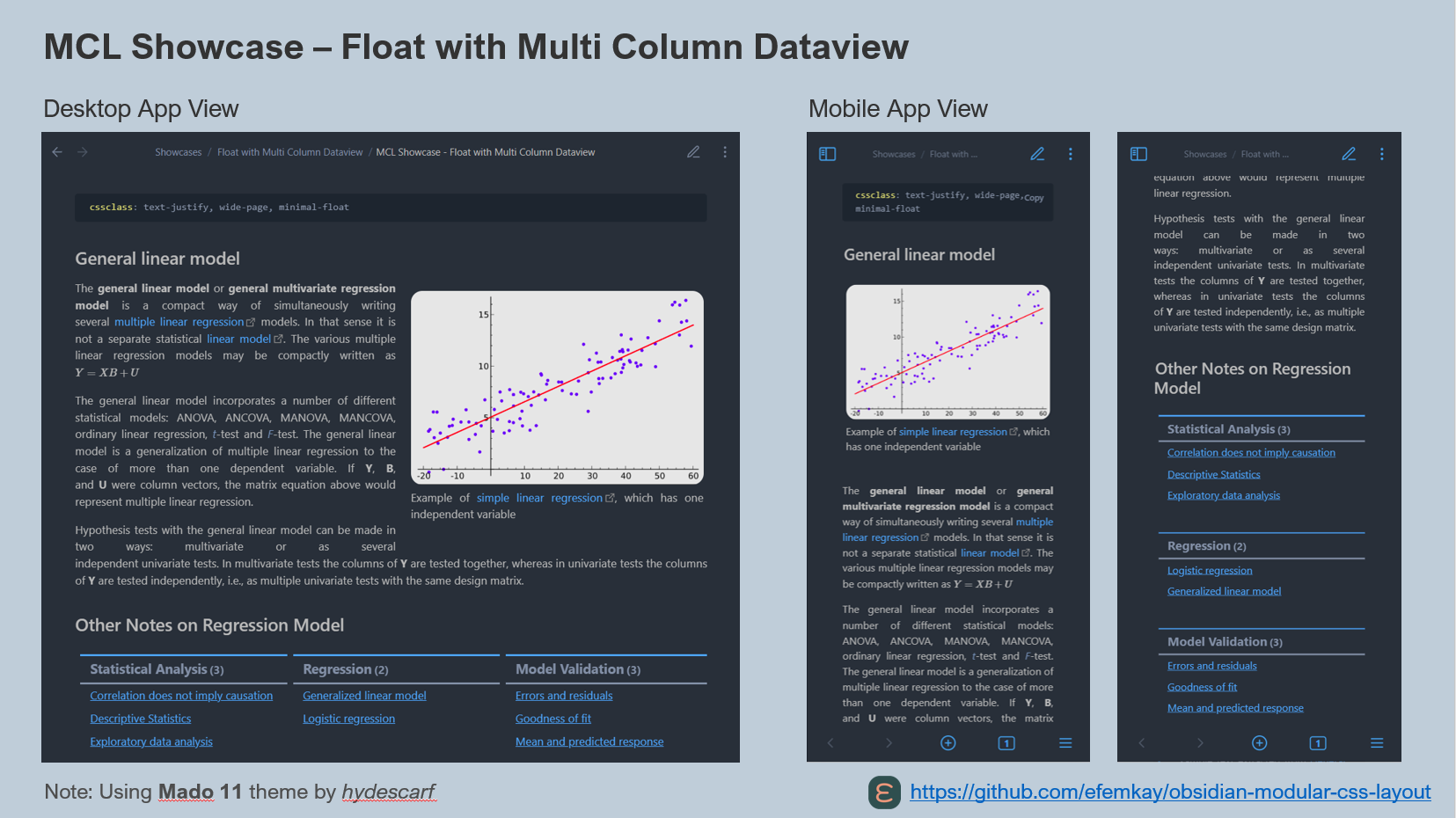#### Screenshot#### Raw Markdown

``````---
cssclass: text-justify, wide-page, minimal-float
---

## General linear model

> [!blank-container|right-medium] Relationship of Data, Information and Intelligence
> ![[Pasted image 20220424085124.png]]
> Example of [simple linear regression](https://en.wikipedia.org/wiki/Simple_linear_regression "Simple linear regression"), which has one independent variable

The **general linear model** or **general multivariate regression model** is a compact way of simultaneously writing several [multiple linear regression](https://en.wikipedia.org/wiki/Multiple_linear_regression "Multiple linear regression") models. In that sense it is not a separate statistical [linear model](https://en.wikipedia.org/wiki/Linear_model "Linear model"). The various multiple linear regression models may be compactly written as \$Y=XB + U\$

The general linear model incorporates a number of different statistical models: ANOVA, ANCOVA, MANOVA, MANCOVA, ordinary linear regression, _t_-test and _F_-test. The general linear model is a generalization of multiple linear regression to the case of more than one dependent variable. If **Y**, **B**, and **U** were column vectors, the matrix equation above would represent multiple linear regression.

Hypothesis tests with the general linear model can be made in two ways: multivariate or as several independent univariate tests. In multivariate tests the columns of **Y** are tested together, whereas in univariate tests the columns of **Y** are tested independently, i.e., as multiple univariate tests with the same design matrix.

## Other Notes on Regression Model
> [!multi-column]
>
> ```dataview
> table without id file.link as "Statistical Analysis"
> from "sample data A"
> ```
>
> ```dataview
> table without id file.link as "Regression"
> from "sample data B"
> ```
>
> ```dataview
> table without id file.link as "Model Validation"
> from "sample data C"
> ```

> sample text extracted from [General linear model - Wikipedia](https://en.wikipedia.org/wiki/General_linear_model) and [Linear regression - Wikipedia](https://en.wikipedia.org/wiki/Linear_regression#Simple_and_multiple_linear_regression)
---
``````